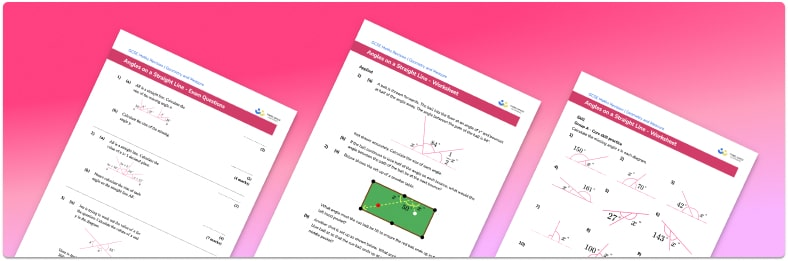# Angles On A Straight Line Worksheet• Section 1 of the angles on a straight line worksheet contains 36 skills-based angles on a straight line questions, in 3 groups to support differentiation
• Section 2 contains 4 applied angles on a straight line questions with a mix of worded problems and deeper problem solving questions
• Section 3 contains 4 foundation and higher level GCSE exam style questions
• Answer keys and a mark scheme for all questions are provided
• Questions follow variation theory with plenty of opportunities for students to work independently at their own level
• All questions created by fully qualified expert secondary maths teachers
• Suitable for GCSE maths revision for AQA, OCR and Edexcel exam boards

• This field is for validation purposes and should be left unchanged.

You can unsubscribe at any time (each email we send will contain an easy way to unsubscribe). To find out more about how we use your data, see our privacy policy.

### Angles on a straight line at a glance

Measuring angles with a protractor on a scale diagram is one way to find the size of unknown angles. However, a more efficient method is to use known angle facts when finding missing angles or calculating angles in 2D shape problems.

Angles on a straight line sum to 180°, so if, for example, we know that acute angle ‘a’ and obtuse angle ‘b’ lie on a straight line, and we know the size of angle ‘a’, we can find the size of angle ‘b’ by subtracting angle ‘a’ from 180°.

A special case of this rule is pairs of angles whose sum is 180° – these are called supplementary angles (this terminology is not required at GCSE, but the idea is useful).

Sometimes a missing angle problem might include a right angle, marked with the ∟ symbol. This can confuse students, so it’s important they recognise the symbol for a right angle, use subtraction from 180° to leave 90°, and then use other information to solve to find the unknown angle.

The fact about angles on a straight line can be extended further to 2D shape problems requiring students to solve an equation to find unknown angles.

Looking forward, students can then progress to additional angles rules worksheets and other geometry worksheets, for example an angles in polygons worksheet or volume and surface area of spheres worksheet.For more teaching and learning support on Geometry our GCSE maths lessons provide step by step support for all GCSE maths concepts.

## Do you have KS4 students who need more focused attention to succeed at GCSE?There will be students in your class who require individual attention to help them succeed in their maths GCSEs. In a class of 30, it’s not always easy to provide.

Help your students feel confident with exam-style questions and the strategies they’ll need to answer them correctly with our dedicated GCSE maths revision programme.

Lessons are selected to provide support where each student needs it most, and specially-trained GCSE maths tutors adapt the pitch and pace of each lesson. This ensures a personalised revision programme that raises grades and boosts confidence.

Find out more